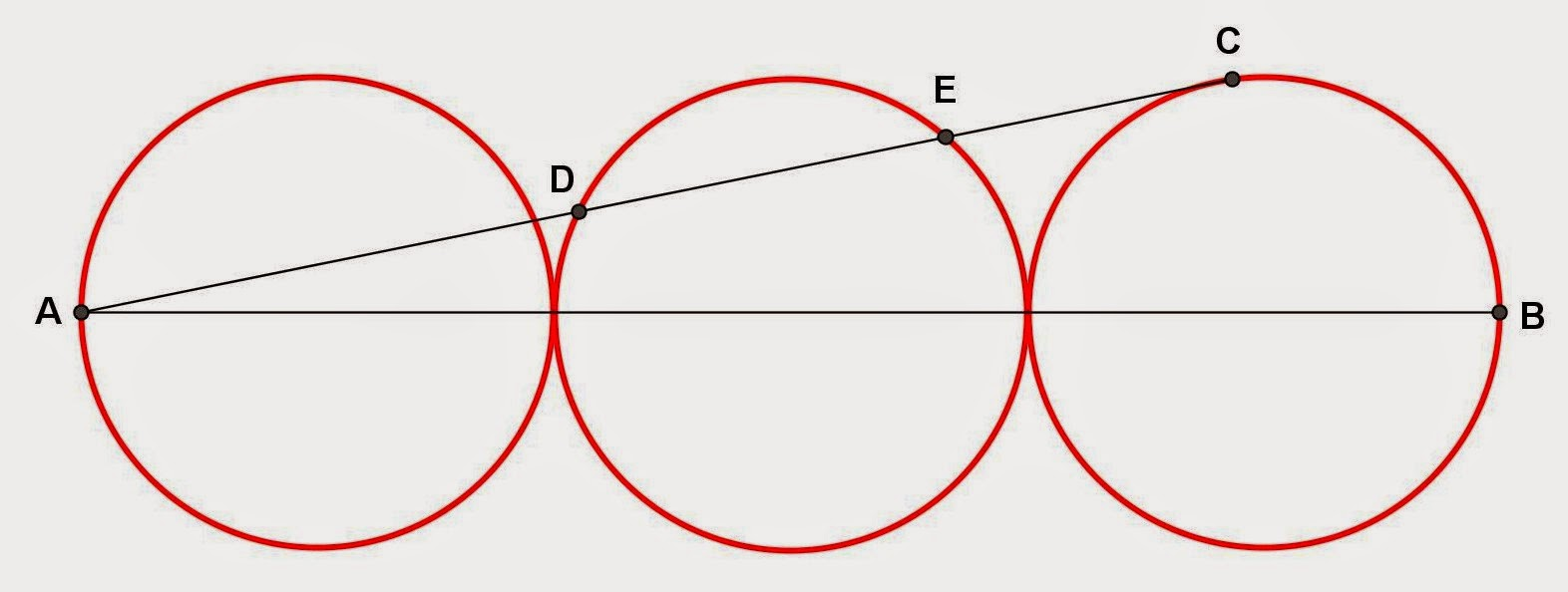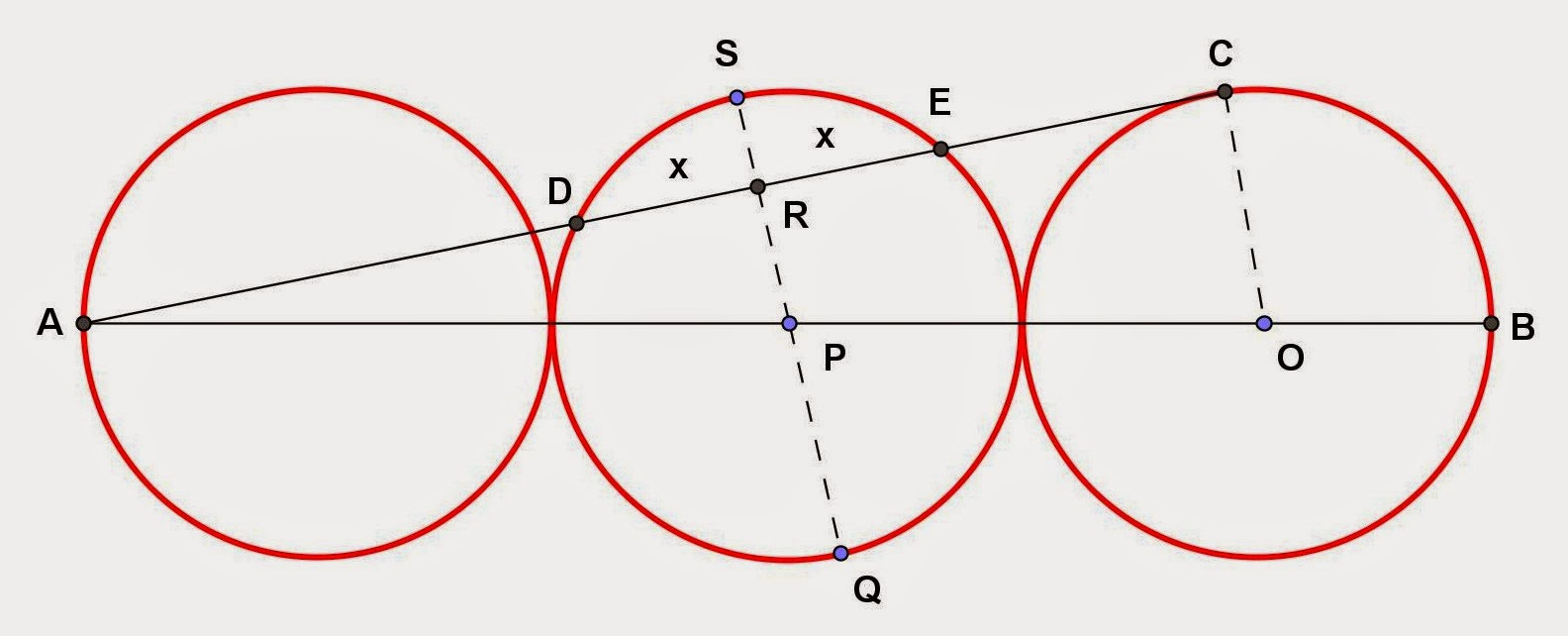Friday, December 26, 2014

Three Tangent Circles, 2

Category: Plane Geometry

"Published in Vacaville, California, USA"

Three tangent circles of radius 10 cm are drawn. All centers lie on the line AB. The tangent AC to the right-hand circle is drawn, intersecting the middle circle at D and E. Find the length of the segment DE.Photo by Math Principles in Everyday Life

Solution:

Consider the given figure abovePhoto by Math Principles in Everyday Life

Since AC is tangent to the third circle at C, then the radius of third circle is perpendicular to AC. Hence, the three lines becomes a right triangle as followsPhoto by Math Principles in Everyday Life

By Pythagorean Theorem, the length of AC is

At the center of second circle, draw a line which is perpendicular to DE. Extend that line at the opposite ends of second circle as followsPhoto by Math Principles in Everyday Life

If SQ is perpendicular to DE and passes thru the center of the circle P, then SQ bisects DE. Since RP is perpendicular to AC and CO is perpendicular to AC, then RP is parallel to CO. Because of this ∆ARP and ∆ACO are similar.

If ∆ARP and ∆ACO are similar, then we can solve for RP by ratio and proportion as follows

If a theorem says "When two chords intersect inside a circle, the product of the segments of one chord equals the product of the segments of the other chord.", then the working equation for the second circle is

Therefore, the length of DE is